# NOW Function

Returns the current date and time

## What is the NOW Function?

The NOW function is categorized under Excel DATE/TIME functions. The function returns the current date and time.

In financial analysis, the NOW function can be useful in preparing various KPI reports. We can use it to display the current date and time, as it will continually update each time the worksheet is refreshed. For example, every time a value is entered or changed, NOW will update the date and time. If we want, we can use F9 to force the worksheet to recalculate and update the value.

### Formula

=NOW()

The NOW function takes no arguments and requires only empty parentheses. The value returned by the function is a serial number representing a particular date and time in Excel.

### How to use the NOW Function in Excel?

As a worksheet function, NOW can be entered as part of a formula in a cell of a worksheet. To understand the uses of the function, let’s consider an example:

#### Example 1

Sometimes when we want to display the current date and time in a worksheet, we can use the NOW function. The date and time will update whenever the worksheet is recalculated or opened.

To do that, we need to just insert the formula =NOW() in the cell where the current date and time should appear.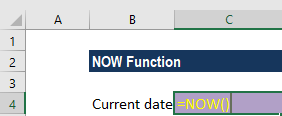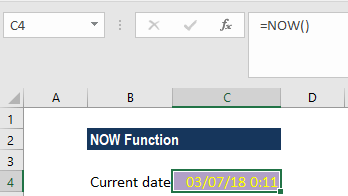#### Example 2

Suppose we want Excel to tell us when our next appointment is due. For this, we will arrange the appointments as shown below: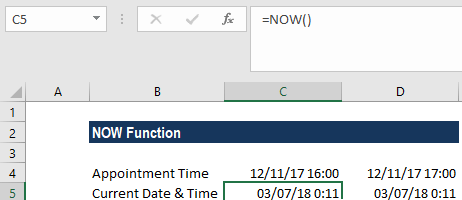For row 5, we used the NOW function as shown below: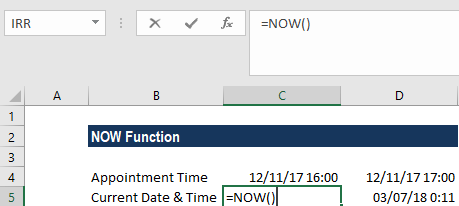Now we will input the formula =IF(C4-C5<1/24,”Due within 1 hr!!!”,”Not due yet”)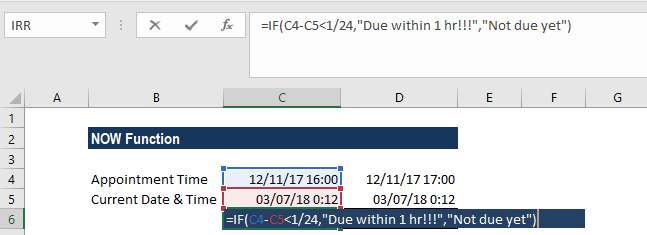We will get the result below: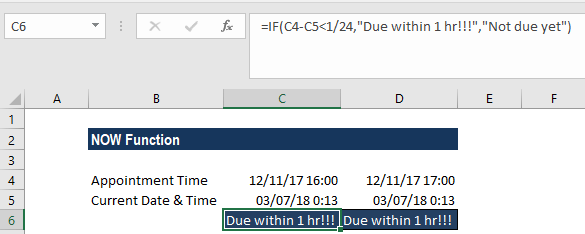In the example above, the current time that is returned by the NOW function is subtracted from the appointment time and the result is compared against the value 1/24, which is the value of 1 hour in Excel. If the current time is less than 1 hour from the appointment time, the alert is displayed. Otherwise, the text “Not yet due” is displayed.

Remember that the above appointment alert will only work if our spreadsheet is continually updated. While the spreadsheet is unused, the content of cells B4 and C4 will stay the same as at the last evaluation.

### A few things to remember about the NOW Function

1. Time values are a portion of a date value and represented by a decimal number (for example, 12:00 PM is represented as 0.5 because it is half of a day).
2. #VALUE! error – Occurs when the given serial_number is not a valid Excel time.
3. If we want to display the date only, we can format the cell with a date format that does not display time, or we can use the TODAY function, which only inserts the date component.
4. If we want to insert the current date and time in such a way that they will not change, we need to use the keyboard shortcut Ctrl + ‘ (press all 3 keys together). The shortcut will insert the current time in a cell as a value that will not automatically change.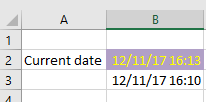If we need to insert both the date and time in a cell, use the keyboard shortcut Ctrl + ; then enter a space character and press Ctrl + Shift + ;

Thanks for reading CFI’s guide to important Excel functions! By taking the time to learn and master these functions, you’ll significantly speed up your financial analysis. To learn more, check out these additional CFI resources:

• Excel Functions for Finance